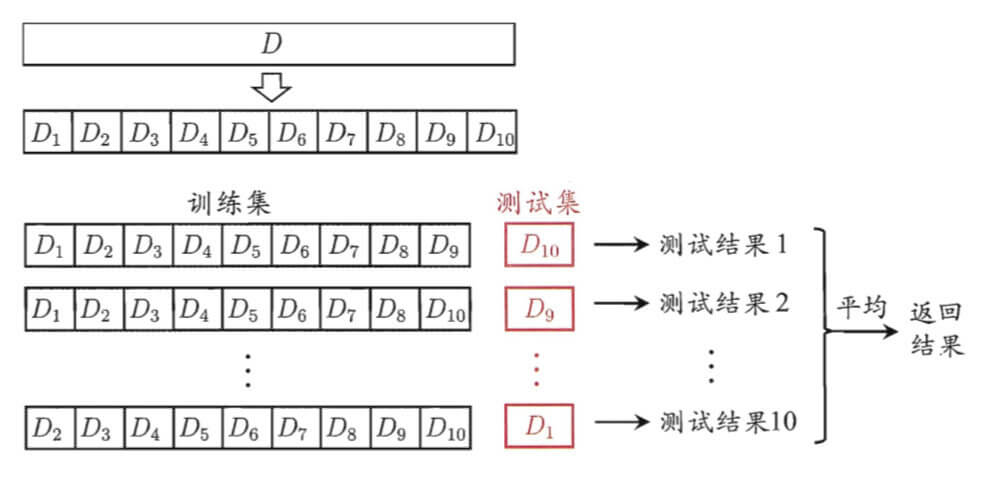# 【机器学习基础】第二课：模型评估方法

## 误差，过拟合，留出法，交叉验证法，自助法

Posted by x-jeff on November 27, 2018

【机器学习基础】系列博客为参考周志华老师的《机器学习》一书，自己所做的读书笔记。

# 1.经验误差与过拟合

• 误差：把学习器的实际预测输出与样本的真实输出之间的差异称为“误差”。
• 训练误差（经验误差）：学习器在训练集上的误差。
• 泛化误差：在新样本上的误差。

❗️针对“模型选择”问题，理想的解决方案当然是对候选模型的泛化误差进行评估（但是在现实任务中，往往还会考虑时间开销、存储开销、可解释性等方面的因素，这里暂且只考虑泛化误差）。

# 2.模型评估方法

⚠️通常以测试集的“测试误差”作为泛化误差的近似。

## 2.1.留出法

“留出法”：直接将数据集D划分为两个互斥的集合，其中一个集合作为训练集S，另一个作为测试集T

## 2.2.交叉验证法

10折交叉验证示意图：“k折交叉验证”：返回平均结果。进一步，p次k折交叉验证，如10次10折交叉验证。

• 优点：留一法使用的训练集与初始数据集相比只少了一个样本，这就使得在绝大多数情况下，留一法中被实际评估的模型与期望评估的用D训练出的模型很相似。因此，留一法的评估结果往往被认为比较准确。
• 缺点：
1. 在训练集比较大时，训练m个模型的计算开销可能是难以忍受的。
2. 留一法的评估结果也未必永远比其他评估方法准确。

## 2.3.自助法

1. 在留出法和交叉验证法中，由于保留了一部分样本用于测试，因此实际评估的模型所使用的训练集比D小，这必然会引入一些因训练样本规模不同而导致的估计偏差。
2. 留一法受训练样本规模变化的影响较小，但计算复杂度又太高了。

“自助法”是以自助采样（又称可重复采样、有放回采样）为基础。

⚠️自助法有两个要点：1.有放回抽样；2.样本量$D=D’$。

$\lim_{m\to\infty}(1-\frac{1}{m})^m=[\lim_{m\to\infty}(1+\frac{1}{-m})^{-m}]^{-1}=\frac{1}{e}\approx0.368$

• 极限是函数当自变量趋向无限大或某一定值时所表现的一种特性。
• 微分是函数在某一点处因变量的增量和自变量增量之间存在的一种特殊关系。

1. $\lim[f(x)\pm g(x)]=\lim{f(x)}\pm\lim{g(x)}$
2. $\lim{c\cdot f(x)}=c\lim{f(x)}$
3. $\lim[f(x)\cdot g(x)]=[\lim{f(x)}]\cdot [\lim{g(x)}]$
4. $\lim{\frac{f(x)}{g(x)}}=\frac{\lim{f(x)}}{\lim{g(x)}}$($\lim{g(x)}\neq 0$)

# 3.调参与最终模型